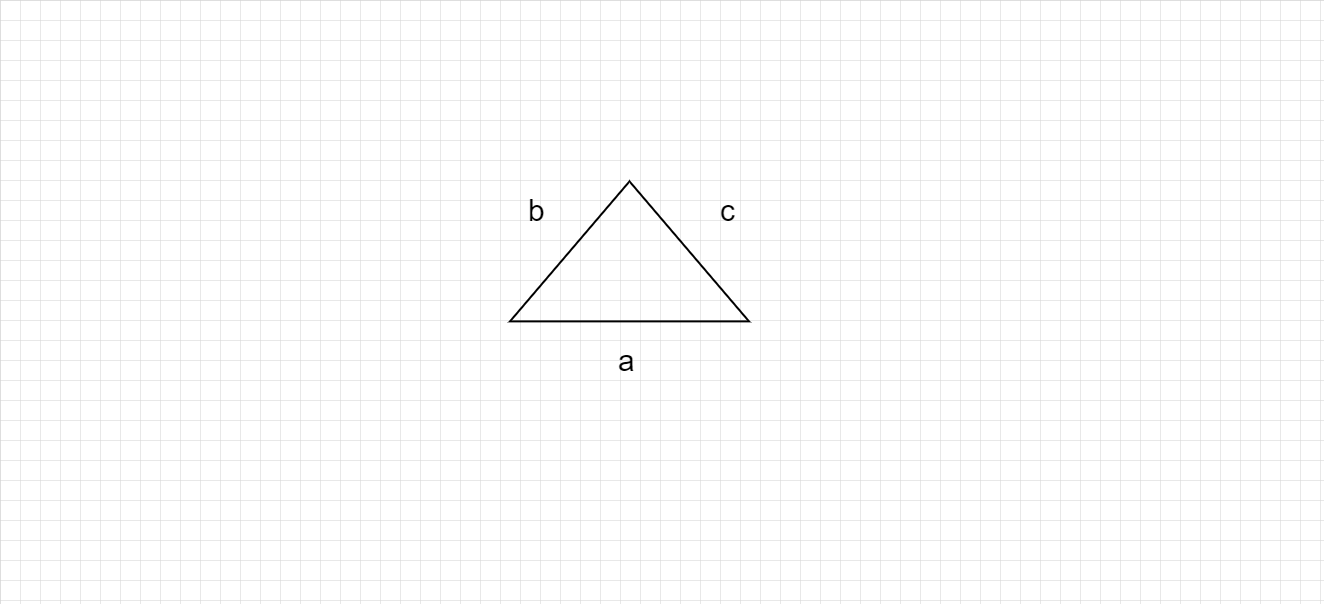# What is perimeter ? How to find the perimeter of triangle ?

Perimeter :

In geometry, perimeter can be defined as the path or the boundary that surrounds a shape. It can also be defined as the length of the outline of a shape.

Perimeter of triangle :

Perimeter of the triangle below with sides of length a units, b units and c units $= (a + b + c)$ units.